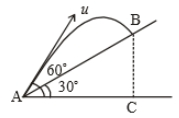A particle is projected at an angle $\theta$ with horizontal with an initital speed u. When it makes an angle $\alpha$ with horizontal, its speed v is-

1. $u\mathrm{cos}\theta$

2.

3. $\frac{u\mathrm{sin}\theta }{\mathrm{sin}\alpha }$

4. $\frac{u\mathrm{cos}\theta }{\mathrm{cos}\alpha }$

Subtopic:  Projectile Motion |
To view explanation, please take trial in the course below.
NEET 2022 - Target Batch - Aryan Raj Singh

Difficulty Level:

A particle is moving along the path y = ${\mathrm{x}}^{2}$ from x = 0 m to x = 2 m. Then the distance traveled by the particle is:

1.  4 m

2.

3.

4.

Subtopic:  Position & Displacement |
To view explanation, please take trial in the course below.
NEET 2022 - Target Batch - Aryan Raj Singh

Difficulty Level:

Six particles situated at the corners of a regular hexagon of side 'a' move at constant speed v. Each particle maintains a direction towards the particle at the next. The time which the particles will take to meet each other is-

1.

2.

3.

4.

Subtopic:  Relative Motion |
To view explanation, please take trial in the course below.
NEET 2022 - Target Batch - Aryan Raj Singh

Difficulty Level:

A body is projected with velocity $20\sqrt{3}$ m/s with an angle of projection 60$°$ with horizontal. Calculate velocity on that point where body makes an angle 30$°$ with the horizontal.

1. 20 m/s

2.

3.

4. 10 m/s

Subtopic:  Projectile Motion |
To view explanation, please take trial in the course below.
NEET 2022 - Target Batch - Aryan Raj Singh

Difficulty Level:

A particle is moving with veocity $\stackrel{\to }{\mathrm{v}}=\mathrm{k}\left(\mathrm{y}\stackrel{^}{\mathrm{i}}+\mathrm{x}\stackrel{^}{\mathrm{j}}\right)$; where k is constant. The general equation for the path is:

1. $\mathrm{y}={\mathrm{x}}^{2}+\mathrm{constant}$

2. ${\mathrm{y}}^{2}={\mathrm{x}}^{2}+\mathrm{constant}$

3. $\mathrm{y}=\mathrm{x}+\mathrm{constant}$

4. xy=constant

Subtopic:  Speed & Velocity |
To view explanation, please take trial in the course below.
NEET 2022 - Target Batch - Aryan Raj Singh

Difficulty Level:

A particle is projected with a velocity u making an angle $\mathrm{\theta }$ with the horizontal. At any instant, its velocity v is at right angles to its initial velocity u; then v is:

1.  u cos $\mathrm{\theta }$

2.  u tan $\mathrm{\theta }$

3.  u cot $\mathrm{\theta }$

4.  u sec $\mathrm{\theta }$

Subtopic:  Projectile Motion |
To view explanation, please take trial in the course below.
NEET 2022 - Target Batch - Aryan Raj Singh

Difficulty Level:

A projectile is given an initial velocity of $\stackrel{^}{i}+2\stackrel{^}{j}$. The cartesian equation of its path is (g = 10 ${\mathrm{ms}}^{-2}$

1. $\mathrm{y}=2\mathrm{x}-5{\mathrm{x}}^{2}$

2. $\mathrm{y}=\mathrm{x}-5{\mathrm{x}}^{2}$

3. $4\mathrm{y}=2\mathrm{x}-5{\mathrm{x}}^{2}$

4. $\mathrm{y}=2\mathrm{x}-25{\mathrm{x}}^{2}$

Subtopic:  Projectile Motion |
To view explanation, please take trial in the course below.
NEET 2022 - Target Batch - Aryan Raj Singh

Difficulty Level:

A ship A is moving westwards with a speed of 10 km ${\mathrm{h}}^{-1}$ and a ship B, 100 km south of A is moving northwards with a speed of 10 km ${\mathrm{h}}^{-1}$. The time after which the distance between them becomes the shortest, is:

1. 5 hr

2. $5\sqrt{2}$ hr

3. $10\sqrt{2}$ hr

4. 0 hr

Subtopic:  Relative Motion |
To view explanation, please take trial in the course below.
NEET 2022 - Target Batch - Aryan Raj Singh

Difficulty Level:

Time taken by the projectile to reach from A to B is t. Then the distance AB is equal to :1. $\frac{ut}{\sqrt{3}}$

2. $\frac{\sqrt{3}ut}{2}$

3. $\sqrt{3}ut$

4. 2ut

Subtopic:  Projectile Motion |
To view explanation, please take trial in the course below.
NEET 2022 - Target Batch - Aryan Raj Singh

Difficulty Level:

A particle projected with kinetic energy ${\mathrm{k}}_{0}$ with an angle of projection $\mathrm{\theta }$. Then the variation of kinetic K with vertical displacement y is

1.  linear

2.  parabolic

3.  hyperbolic

4.  periodic

Subtopic:  Projectile Motion |
To view explanation, please take trial in the course below.
NEET 2022 - Target Batch - Aryan Raj Singh

Difficulty Level: